﻿ Temperature-Dependent Heat Source (Transient Analysis)Examples | Product | Murata Software Co., Ltd.# Example17Temperature-Dependent Heat Source (Transient Analysis)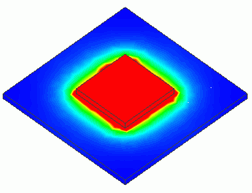### General

• The model is the same as Exercise 8: A heat source is placed on a substrate, and there is a forced air flow for cooling in parallel to the substrate. The heat radiation is analyzed under the transient condition.
The heat source is temperature-dependent.

• The heat transfer coefficient is acquired manually.
To acquire it automatically, see “Ex.1 of Simple Fluid-Thermal Analysis”.

• The temperature distribution and the heat flux vectors are solved.

• Unless specified in the list below, the default conditions will be applied.

### Analysis Space

 Item Settings Analysis Space 3D Model unit mm

### Analysis Conditions

 Item Settings Solvers Thermal Analysis [Watt] Analysis Type Transient analysis Options N/A

The transient analysis is set up in transient tab as follows. The total number of steps is 20. The time step is 30 second.

Therefore, the temperature distributions for 600 seconds are solved.

Tab

Setting Item

Settings

Transient analysis

Table

 Number Calculation steps Output steps Time step [s] 1 20 1 30

Initial Temperature

25[deg]

### Model

The same as Exercise 7. The material properties and the boundary conditions are the same as well.

The substrate (VOL1) and the heat source (VOL2) are created as solid body box, and the heat source is defined in the body attribute of VOL2.

The heat transfer coefficients for the top and bottom faces of the substrate and the top face of the heat source are calculated based on the simplified equation.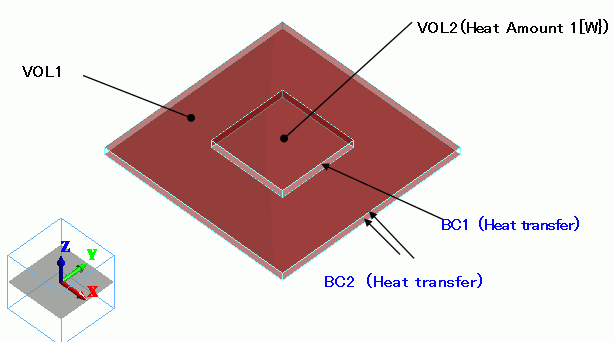### Body Attributes and Materials

 Body Number/Type Body Attribute Name Material Name 0/Solid VOL1 006_Glass_epoxy * 1/Solid VOL2 001_Alumina *

* Available from the Material DB

The heat source of VOL2 is set up as follows.

 Body Attribute Name Tab Settings VOL2 Heat Source Heat density Temperature Dependency: Yes

Heat source is specified by heat density in the analyses where temperature-dependent heating materials are involved.

On Exercise 8, we set 1[w] for the heat source of VOL2. As the volume of VOL2 is 800*10^-9[m^3], the heat density is equal to

1.25 x 10^6 [W/m^3].

This is the value for 25[deg], so

P(25) = 1.25 x 10^6 [W/m^3]

in the Arrhenius equation which is given by the following:

P(T) = 1.25 x 10^6 * exp(-0.15/(k*(T+273))) / exp (-0.15/(k*(25+273)))

where 0.15[eV] is the activation energy and k is the Boltzmann constant.

 Body Attribute Name Item Settings VOL2 Nonlinearity Table Select “Smooth interpolation”

 Temperature [degC] Heat Source x10^6[W/m^3] Temperature [degC] Heat Source x10^6[W/m^3] Temperature [degC] Heat Source x10^6[W/m^3] 25 1.25 155 7.365383041 605 59.18105993 35 1.51094343 205 11.26925562 655 65.85048973 45 1.804711948 255 15.90794507 705 72.47585198 55 2.132370783 305 21.15582761 755 79.02738627 65 2.494768691 355 26.88623825 805 85.48214754 75 2.892545312 405 32.98193112 855 91.82276684 85 3.326141169 455 39.339762 905 98.03640656 95 3.795809582 505 45.87196227 955 104.1138907 105 4.301629871 555 52.5055812 1005 110.0489868

Heat density’s temperature plot is shown below.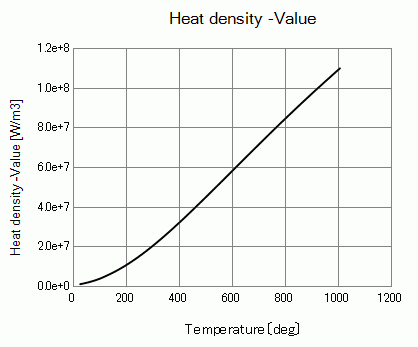### Boundary Conditions

The heat transfer coefficients for the forced convection are calculated as follows. The equation is given in Exercise 8: Heat Radiation by Forced Convection (Transient Analysis).

For the details, please refer to the Heat Transfer Coefficient for Forced Convection

To acquire it automatically, see “Ex.1 of Simple Fluid-Thermal Analysis”.

 Boundary Condition Name/Topology Tab Boundary Condition Type Settings BC1/Face Thermal Heat Transfer/Ambient Radiation Heat transfer coefficient: 17.26[W/m2/deg] Room Temperature : 25[deg] BC2/Face Thermal Heat Transfer/Ambient Radiation Heat transfer coefficient: 27.3[W/m2/deg] Room Temperature : 25[deg]

### Results

The temperature distributions for Exercise 8 (right figures) and this exercise (left figures) are shown below for the elapsed time of 60, 300 and 600 seconds.

The unit of the color scale is [deg].

At Minimum/Maximum Value on the Contour tab of [Graphics Setup], deselect “Automatic” and set 25 => 150.

There is almost no difference in 60 seconds.

However, it becomes obvious that the temperature increases much higher than Exercise 8 in 300 and 450 seconds.

##### In 60 seconds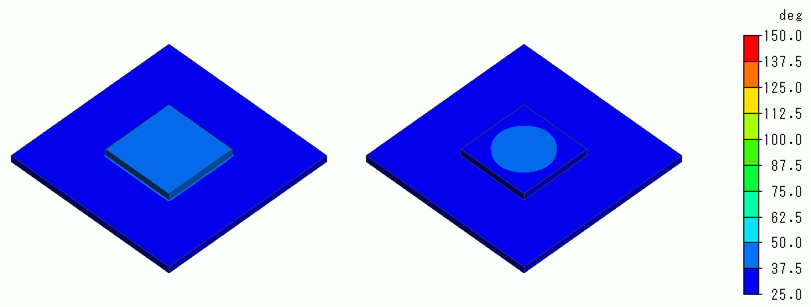##### In 300 seconds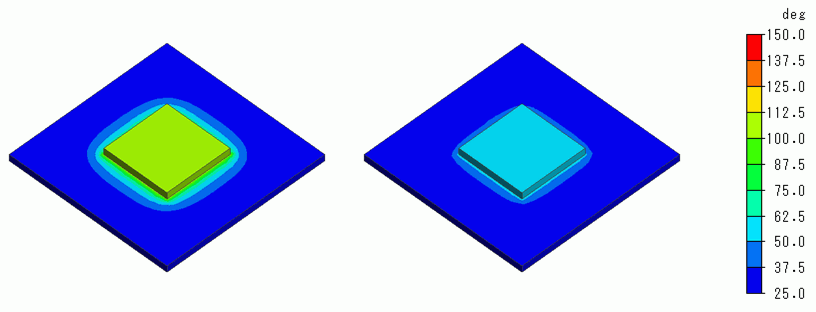##### In 450 seconds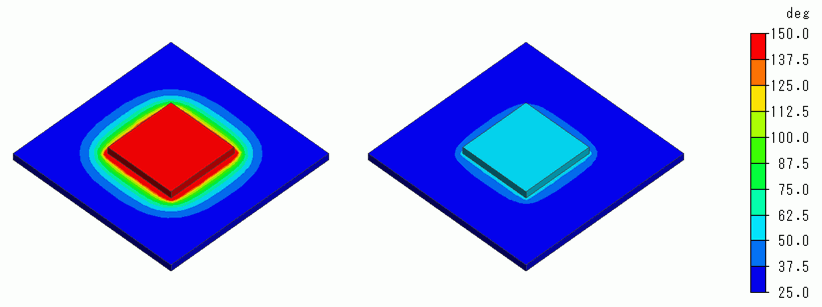The temperature vs. time is plotted below.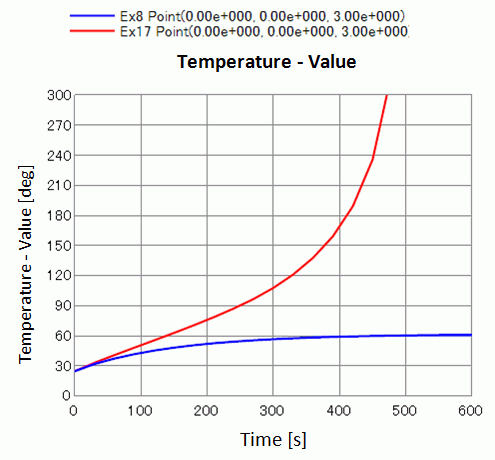﻿